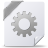# Solidworks and Matlab / Simulink simulation

In this tutorial you will see how we can make integration of Solidworks and Matlab/ Simulink using the SimMechanics Link. We will make an example of Simulink simulation using a Solidworks 3D model. Below the video you can find and download the 3D model and the Simulink model of the example.

Solidworks Model .STEP file:...

Solidworks Model Solidworks assembly and parts files: